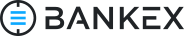Young plasma developer decided to make a plasma contract. He wants to be to the first one who will launch plasma token to the moon.

As a first step, he needs to sign a contract with a first real customer that wants to see that plasma can supports ERC223 tokens.

He works on the demo, but probably his implementation that listed below has a vulnerability. Help him to find it.

Your goal is to assign true to the "contract_status" variable

contract_status == true;

Your smart contract is at 0ceqiccnoijwncoiwncoiwncb on Rinkeby network
```library SafeMath {

/**
* @dev Multiplies two numbers, throws on overflow.
*/
function mul(uint256 a, uint256 b) internal pure returns (uint256) {
if (a == 0) {
return 0;
}
uint256 c = a * b;
assert(c / a == b);
return c;
}

/**
* @dev Integer division of two numbers, truncating the quotient.
*/
function div(uint256 a, uint256 b) internal pure returns (uint256) {
// assert(b > 0); // Solidity automatically throws when dividing by 0
uint256 c = a / b;
// assert(a == b * c + a % b); // There is no case in which this doesn't hold
return c;
}

/**
* @dev Substracts two numbers, throws on overflow (i.e. if subtrahend is greater than minuend).
*/
function sub(uint256 a, uint256 b) internal pure returns (uint256) {
assert(b <= a);
return a - b;
}

/**
* @dev Adds two numbers, throws on overflow.
*/
function add(uint256 a, uint256 b) internal pure returns (uint256) {
uint256 c = a + b;
assert(c >= a);
return c;
}
}

contract ERC223Interface {
uint public totalSupply;

function balanceOf(address who) constant returns (uint);

function transfer(address to, uint value, bytes data);

}

contract ERC223Token is ERC223Interface {
using SafeMath for uint;

mapping(address => uint) balances; // List of user balances.

/**
* @dev Transfer the specified amount of tokens to the specified address.
*      Invokes the `tokenFallback` function if the recipient is a contract.
*      The token transfer fails if the recipient is a contract
*      but does not implement the `tokenFallback` function
*      or the fallback function to receive funds.
*
* @param _value Amount of tokens that will be transferred.
*/
function transfer(address _to, uint _value, bytes _data) {
// Standard function transfer similar to ERC20 transfer with no _data .
// Added due to backwards compatibility reasons .
uint codeLength;

assembly {
// Retrieve the size of the code on target address, this needs assembly .
codeLength := extcodesize(_to)
}

balances[msg.sender] = balances[msg.sender].sub(_value);
if (codeLength > 0) {
}
emit Transfer(msg.sender, _to, _value, _data);
}

/**
* @dev Transfer the specified amount of tokens to the specified address.
*      This function works the same with the previous one
*      but doesn't contain `_data` param.
*      Added due to backwards compatibility reasons.
*
* @param _value Amount of tokens that will be transferred.
*/
function transfer(address _to, uint _value) {
uint codeLength;
bytes memory empty;

assembly {
// Retrieve the size of the code on target address, this needs assembly .
codeLength := extcodesize(_to)
}

balances[msg.sender] = balances[msg.sender].sub(_value);
if (codeLength > 0) {
}
emit Transfer(msg.sender, _to, _value, empty);
}

/**
* @dev Returns balance of the `_owner`.
*
* @param _owner   The address whose balance will be returned.
* @return balance Balance of the `_owner`.
*/
function balanceOf(address _owner) constant returns (uint balance) {
return balances[_owner];
}
}

contract ERC223ReceivingContract {
/**
* @dev Standard ERC223 function that will handle incoming token transfers.
*
* @param _from  Token sender address.
* @param _value Amount of tokens.
*/
function tokenFallback(address _from, uint _value, bytes _data);
}

contract PlasmaChain is ERC223ReceivingContract, ERC223Token {

bool private contract_status;

// possibly multi assets in future

constructor () {
totalSupply = 10000;
balances[this] = totalSupply;
}

balances[msg.sender] = 1000;
}

function Deposit(uint sum) {
transfer(this, sum);
sentSum[msg.sender] = sum;
}

uint codeLength;
bytes memory empty;

assembly {
// Retrieve the size of the code on target address, this needs assembly .
codeLength := extcodesize(_to)
}

balances[_from] = balances[_from].sub(_value);
if (codeLength > 0) {
}
emit Transfer(_from, _to, _value, empty);
return true;
}

function WithDraw() {

transferFrom(this, msg.sender, sentSum[msg.sender]);

sentSum[msg.sender] = 0;

if (balances[this] == 0) {
solved();
}
}

function tokenFallback(address _from, uint _value, bytes _data) {}

function() {
revert();
}

function status() external view returns (bool) {
return contract_status;
}

function solved() private {
contract_status = true;
}

}
```